# Three.js to achieve various filter effects

2021-08-26 20:10:10 Alaso

# Preface

stay three.js Achieve various filter effects in , You need to use custom shader materials `ShaderMaterial`.

Basic knowledge of shaders , We are use three.js Write a 3D The earth This paper introduces . Here you can directly use custom shaders .

# Filter Basics

`WEBGL` The color representation in is RGBA, The color value of each pixel is determined by R、G、B、A The four values decision , The value of each component is in the range of 【0,1】 The floating point number between , for example , Black is （0.0, 0.0, 0.0, 1.0）, White is （1.0, 1.0, 1.0, 1.0）.

`three.js` Encapsulates the `WEBGL` Many implementation details of , Let's develop 3D Applications become simple , It also opens up custom shader materials `ShaderMaterial`, Let developers operate pixels freely , Realize various customized effects .

for example , By customizing shaders , We can manually modify the four component values of each pixel color value . Let's take the earth map as an example .

We use it ShaderMaterial Create an earth , The code is as follows .

``````// Vertex shader code snippet （ When creating shader materials below , Pass in as a parameter ）
varying vec2 v_Uv;    // Vertex texture coordinates
void main () {
v_Uv = uv;
gl_Position = projectionMatrix * viewMatrix * modelMatrix * vec4( position, 1.0 );
}
`

// Fragment shader code （ When creating shader materials below , Pass in as a parameter ）
uniform sampler2D e_Texture;     // texture image
varying vec2 v_Uv;               // Slice texture coordinates
void main () {
gl_FragColor = texture2D( e_Texture, v_Uv );  // Extract the color value of texture image （ Striatin ）, And assign it to gl_FragColor
}
`

// Load the mapped texture image

uniforms: {
e_Texture: {
value: earthTexture   // texture image
}
},
})

// Create an aggregate
var geometry = new THREE.SphereGeometry(20,30,30)

// Create objects
var sphere = new THREE.Mesh(geometry, material)

// Add objects to the scene
Copy code ``````

In the above fragment shader code , We go through `texture2D function ` Extract the color value of a point in the texture image （ Striatin ）, The return value of this function is a four-dimensional vector , Represent the R、G、B、A Four components .

After obtaining the texture element , Assign it to `gl_FragColor`, Used to specify the color of the slice .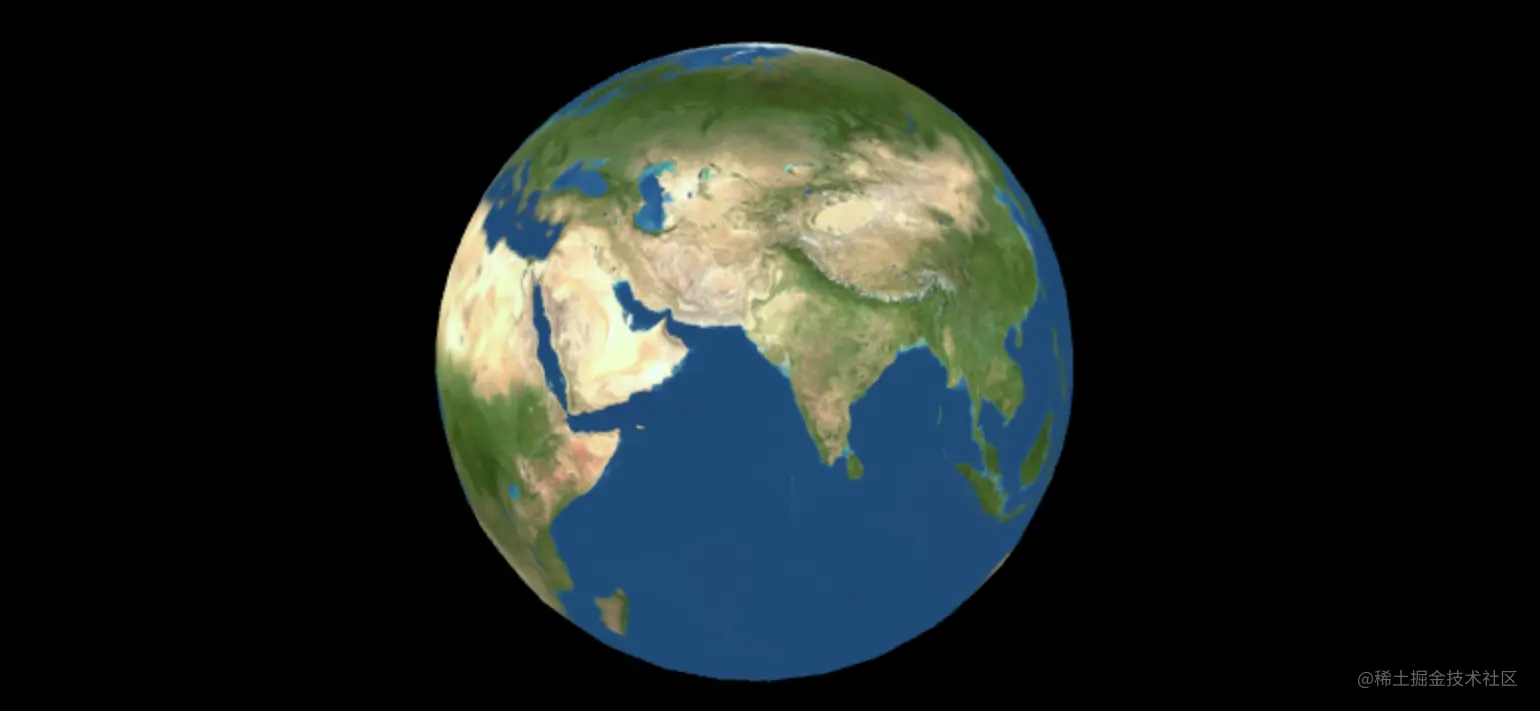## RGBA Component operation

Actually , After we get striatin , You can do it first R、G、B、A These four components do some operations , Assigned to it again `gl_FragColor`.

for example , Put the green of each grain element （G） Set the weight to 0, Modify the slice shader code as follows ：

``````var FSHADER_SOURCE =
`uniform sampler2D e_Texture;
varying vec2 v_Uv;
void main () {
vec4 textureColor = texture2D( e_Texture, v_Uv );
float green = textureColor.g * 0.0;     // Modify the green color of the color value （G） The weight is 0
gl_FragColor = vec4(textureColor.r, green, textureColor.b, 1.0);
}`
Copy code ``````

The effect is as follows ：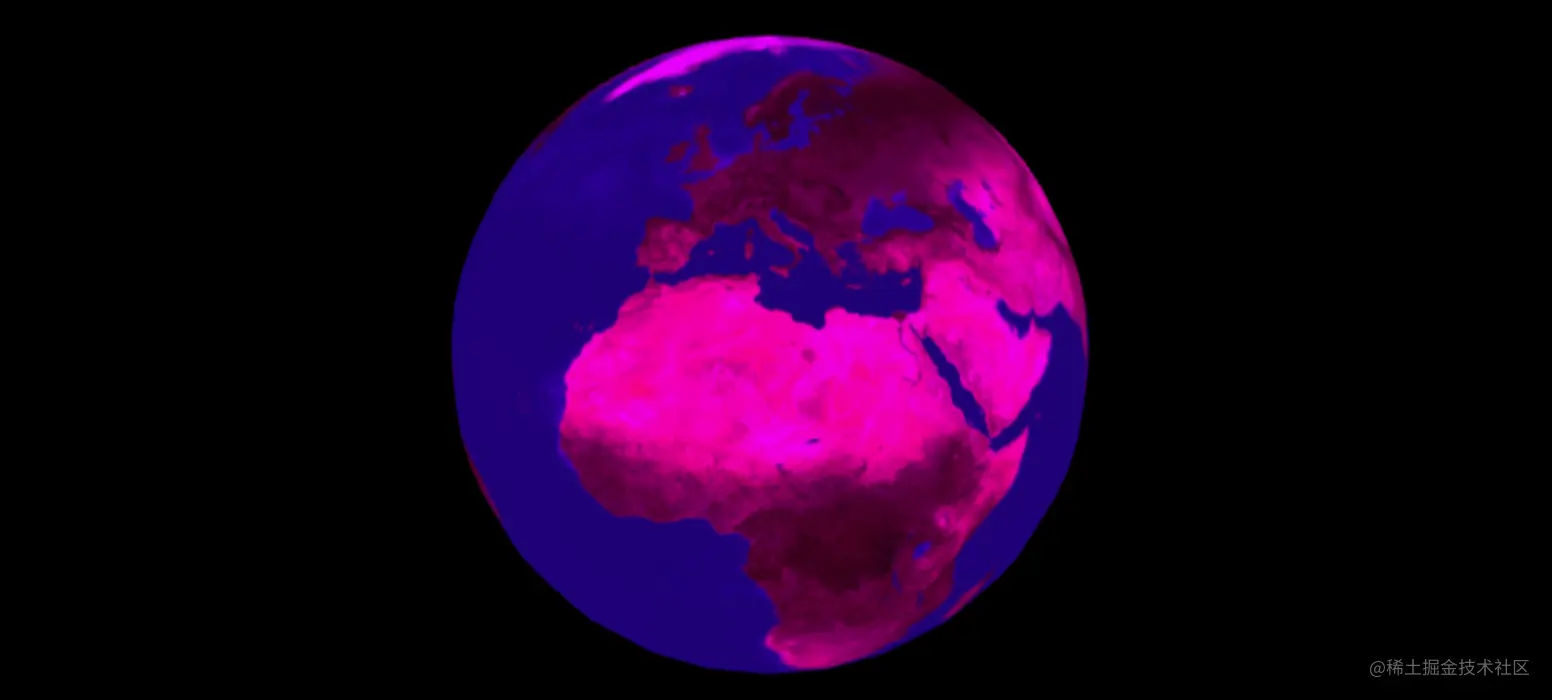see , Does it feel like a filter .

In fact, the essence of the filter , Is to modify the color value of each pixel , A little bit more specific , Is to modify R、G、B、A These four component values .

Let's implement a common gray filter .

# Gray filter principle and matrix implementation

## Basic principle and implementation

Principle of gray scale , Simply put, it is to turn a color picture into a gray picture .

There are three common gray level algorithms ：

• Maximum method
• Average method
• Weighted average method

The next thing to practice is , Weighted average method .

The specific implementation idea is , Let's start with the of each pixel of the picture R、G、B The component values are weighted average , This value is then used as a new value for each pixel R、G、B Component value , The formula is as follows ：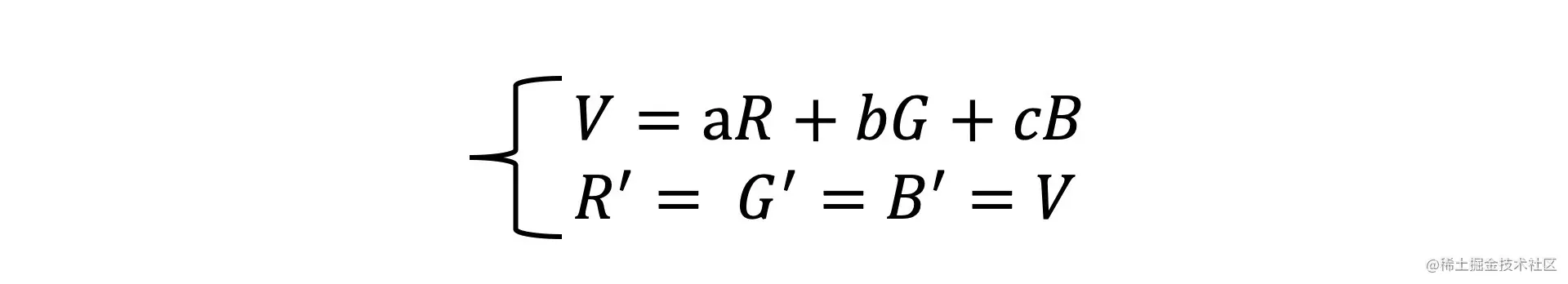among R、G、B It's from the original picture R、G、B The color value of the channel ,V Is the weighted average color value ,a、b、c Is the weighting factor , Satisfy (a + b + c) = 1.

Based on the above principles , Let's make it happen . Modify chip shader code ：

``````var FSHADER_SOURCE =
`uniform sampler2D e_Texture;
varying vec2 v_Uv;
void main () {
vec4 textureColor = texture2D( e_Texture, v_Uv );
// Calculate the weighted average
float w_a = textureColor.r * 0.3 + textureColor.g * 0.6 + textureColor.b * 0.1;
gl_FragColor = vec4(w_a, w_a, w_a, 1.0);
}`
Copy code ``````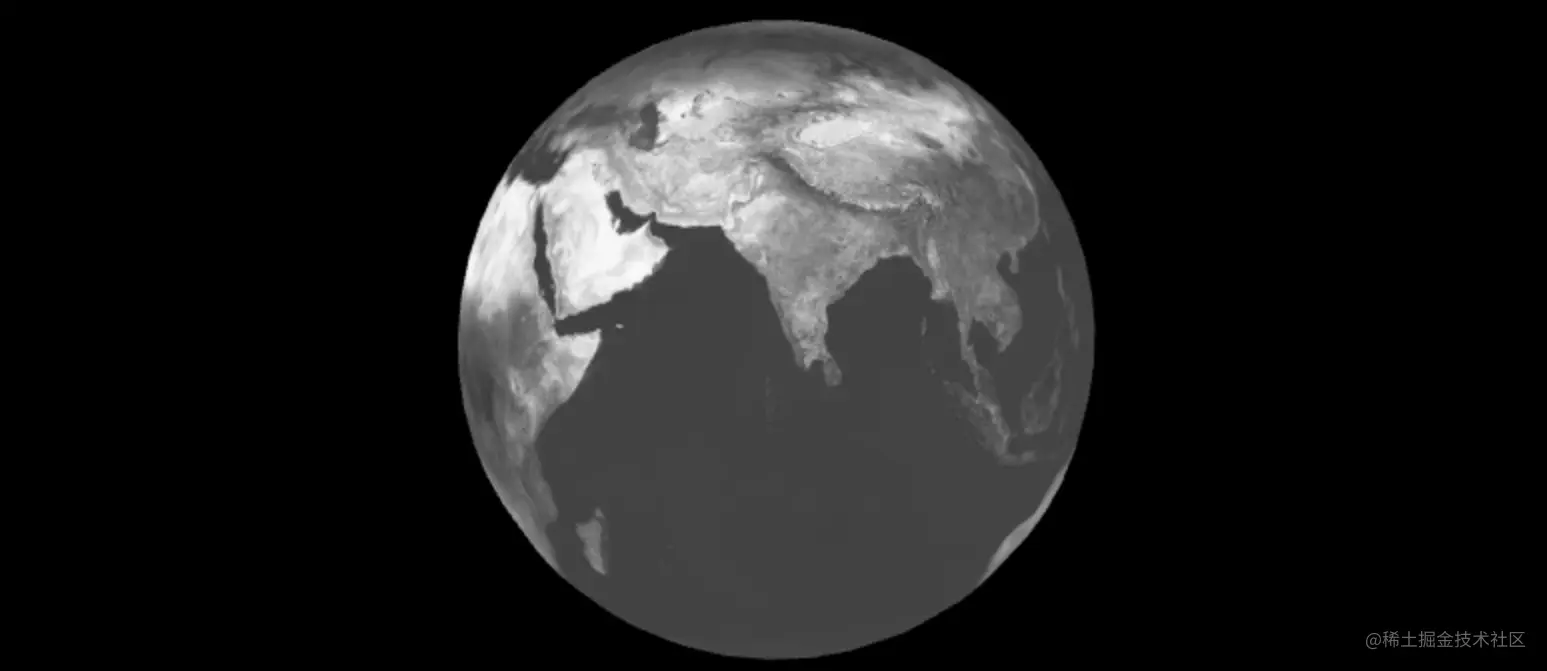The weighting coefficients here are a = 0.3, b = 0.6, c = 0.1, Because human vision is right R、G、B The sensitivity of the three colors is different , So the weights of the three color values are different , Generally speaking, green is the highest , Red is next , Blue minimum . You can change it to other values , Look at the effect .

## Matrix implementation

If you want to adjust the weighting coefficient or implement other filters , You have to modify the slice shader code again . It's too much trouble .

Next, we'll use the matrix to optimize the code .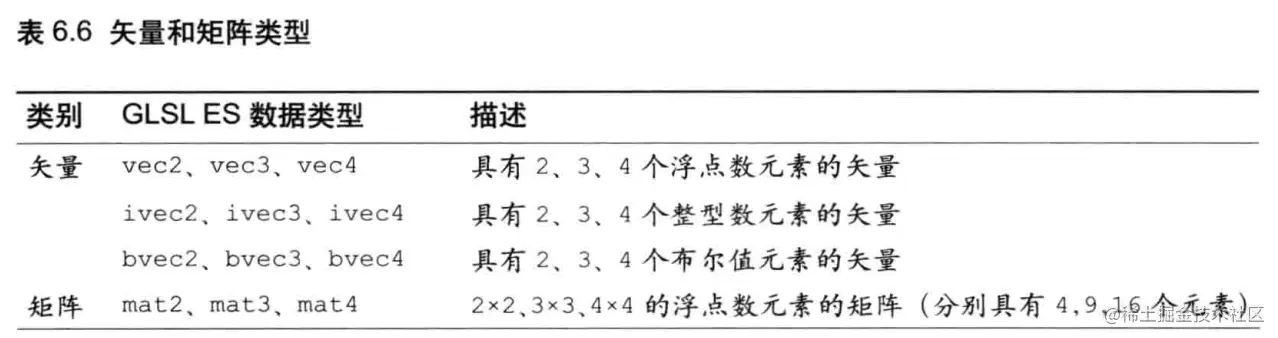because GLSL ES The matrix in is at most four-dimensional , therefore , Here's the pair R、G、B Matrix transformation of three quantities , transparency alpha Set separately .

js The code is modified as follows ：

``````// Shader material
uniforms: {
e_Texture: {
value: earthTexture
},
t_Matrix: {
value: grayMatrix    // The transformation matrix consists of js Pass in
}
},
})
Copy code ``````

The code of the slice shader is also modified accordingly ：

``````var FSHADER_SOURCE =
`uniform sampler2D e_Texture;
uniform mat4 t_Matrix;     // Receive transformation matrix
varying vec2 v_Uv;
void main () {
vec4 textureColor = texture2D( e_Texture, v_Uv );
// The transformation matrix is multiplied by  vec4(R,G,B,1)    --->vec4(R,G,B,1)  Is homogeneous coordinates , It was n The vector of a dimension uses a n+1 Dimension vector
//vec4(R,G,B,1) The fourth component is not transparency
vec4 transColor = t_Matrix * vec4(textureColor.r, textureColor.g, textureColor.b, 1.0);
// Set transparency
transColor.a = 1.0;
gl_FragColor = transColor;
}`
Copy code ``````

According to the multiplication of matrix and vector , The transformation matrix of the gray filter should be as follows ：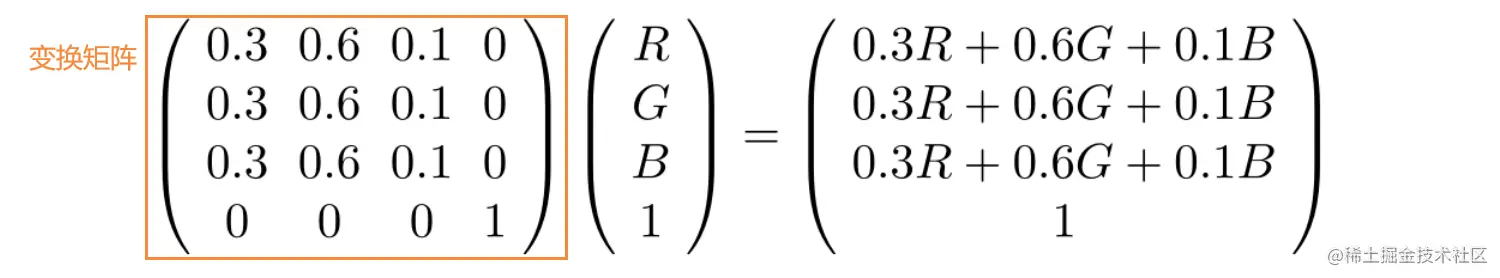But in js The matrix passed in is as follows ,OpenGL API The accepted matrix requirements are ` Column main order ` Of , If one OpenGL Our application uses ` Row main sequence ` Matrix , So you're passing the matrix to OpenGL API front , It needs to be converted to ` Column main order `. We'll manually transpose it here .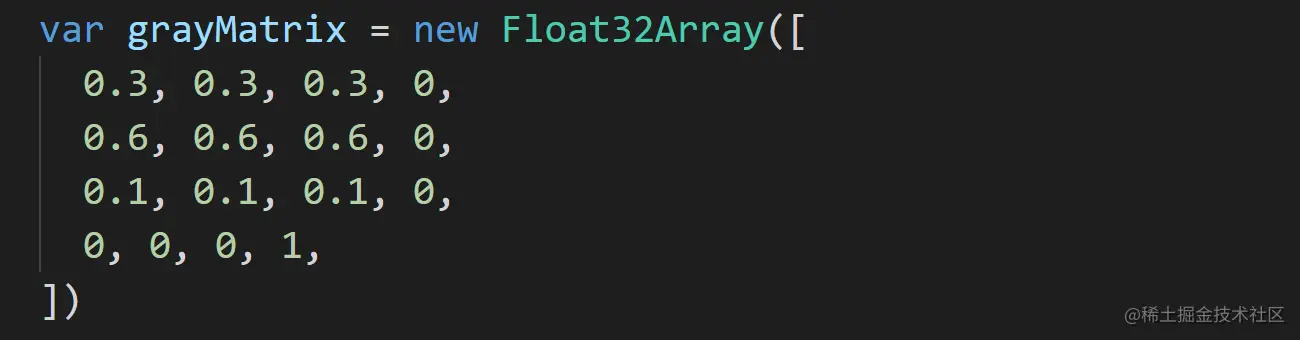Reference material ：OpenGL Row principal order and column principal order of matrix in

Use matrix , It can be well abstracted and reused . A matrix represents a transformation , Just multiply by this matrix , It means doing the same transformation , And when you want to fix some kind of transformation , Just modify its corresponding transformation matrix .

# Realization of several filter effects

Achieve the following filter effects , In fact, several different matrices are used .（ It is worth noting that , The following transformation matrices are also transposed ` Column main order ` matrix . To deduce, please transpose the following matrix back to ` Row main sequence `

## Reverse color

Realize reverse color , Namely R、G、B Each component pair `1` Take complement .

Transformation matrix ：

``````var oppositeMatrix = new Float32Array([
-1, 0, 0, 0,
0, -1, 0, 0,
0, 0, -1, 0,
1, 1, 1, 1
])
Copy code ``````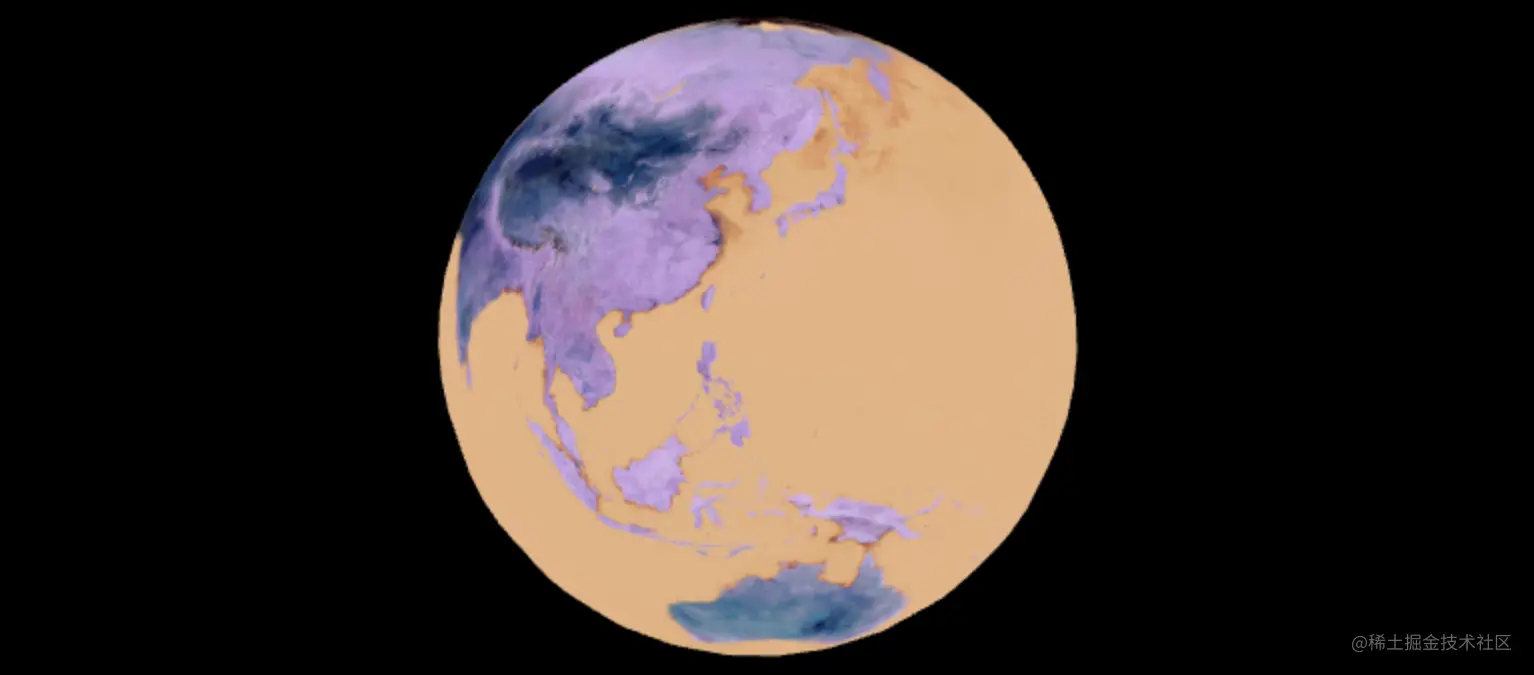## brightness

R、G、B Each component is multiplied by a value `p`; p < 1 Dimming ,p = 1 Primary colors , p > 1 Dimming .

Transformation matrix ：（ Pass in the parameter p, Dynamically generate transformation matrix ）

``````function genBrightMatrix (p) {
return new Float32Array([
p, 0, 0, 0,
0, p, 0, 0,
0, 0, p, 0,
0, 0, 0, 1
])
}
Copy code ``````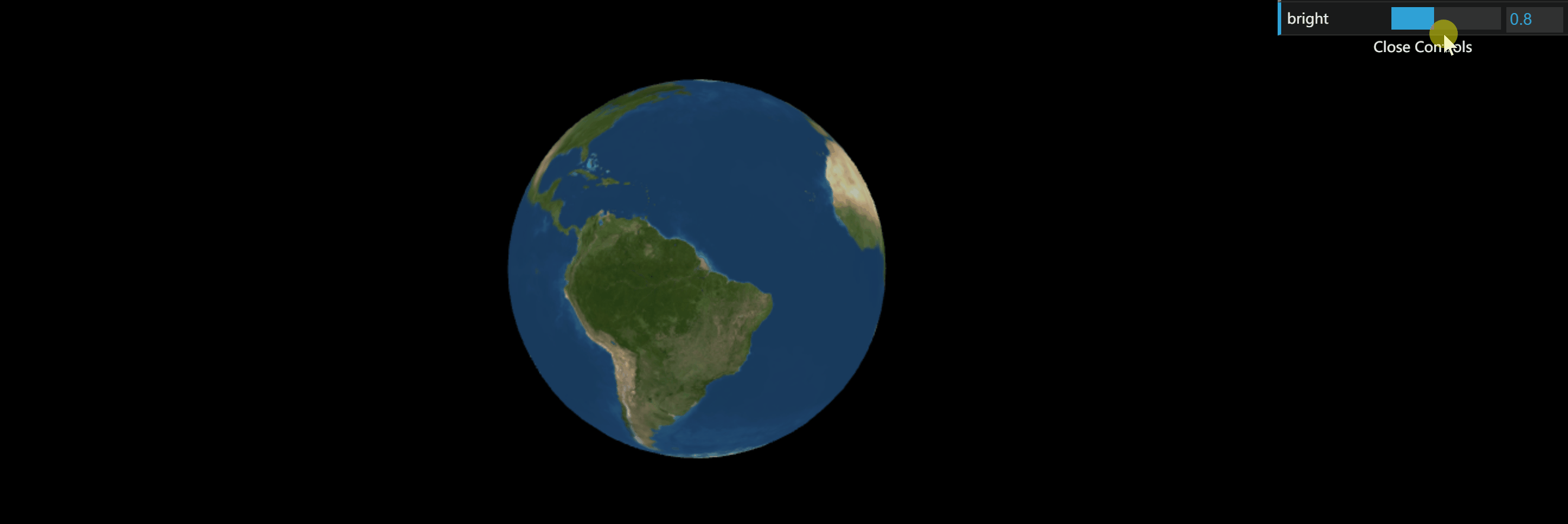## Contrast

R、G、B Each component is multiplied by a value `p`, And then add `0.5 * (1 - p)`; p = 1 Primary colors , p < 1 Reduce contrast ,p > 1 Enhance contrast

Matrix based implementation ：（ Pass in the parameter p, Dynamically generate transformation matrix ）

``````function genContrastMatrix (p) {
var d = 0.5 * (1 - p)
return new Float32Array([
p, 0, 0, 0,
0, p, 0, 0,
0, 0, p, 0,
d, d, d, 1
])
}
Copy code ``````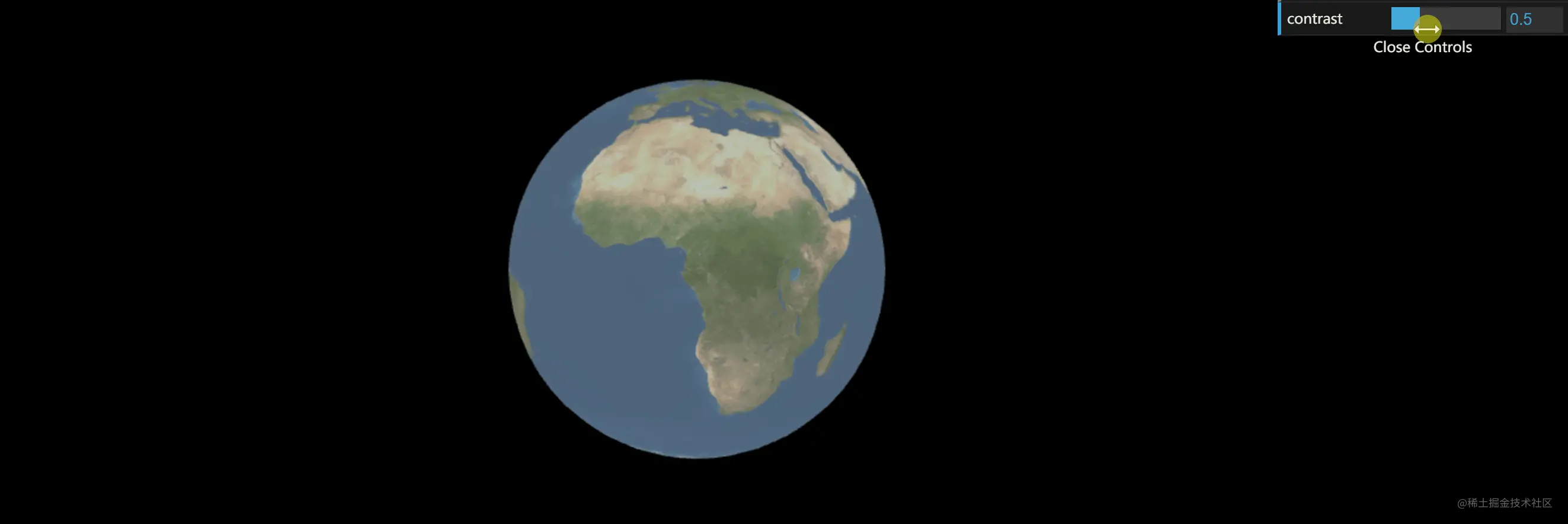## saturation

Pass in the parameter p, The calculation method is as follows .p = 0 Completely grayed out ,p = 1 Primary colors ,p > 1 Enhance saturation .

``````function genSaturateMatrix (p) {
var rWeight = 0.3 * (1 - p)
var gWeight = 0.6 * (1 - p)
var bWeight = 0.1 * (1 - p)
return new Float32Array([
rWeight + p, rWeight, rWeight, 0,
gWeight, gWeight + p, gWeight, 0,
bWeight, bWeight, bWeight + p, 0,
0, 0, 0, 1
])
}
Copy code ``````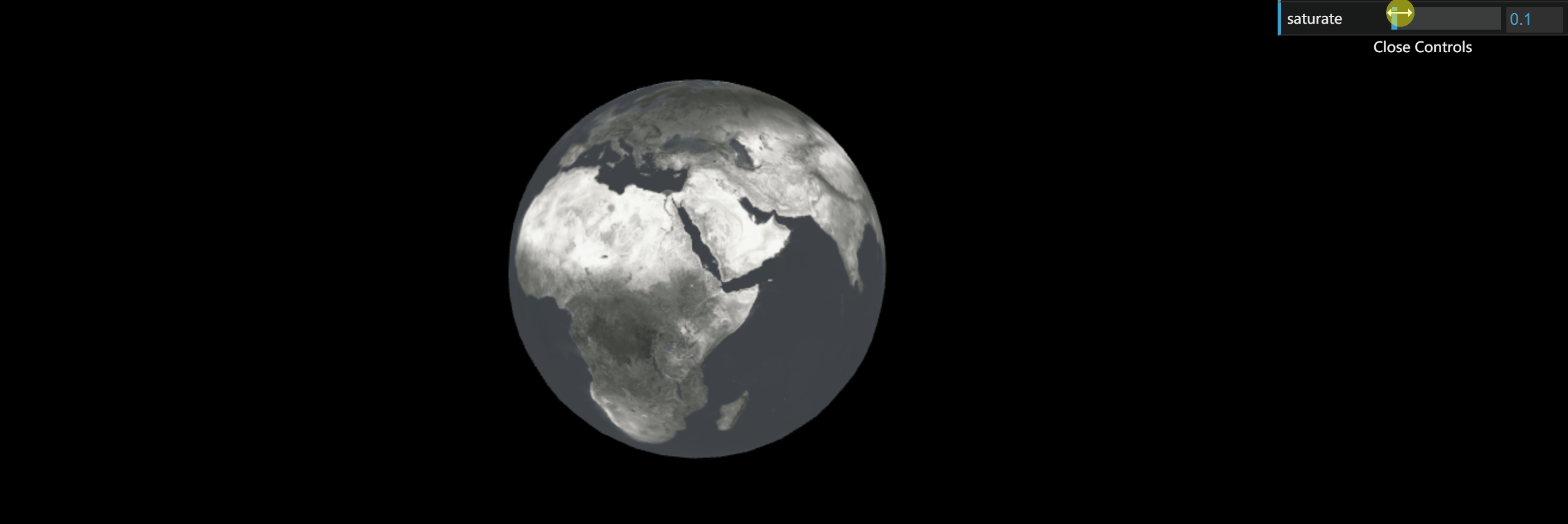# Reference resources

《 Learn visualization from the shadow of the moon 》